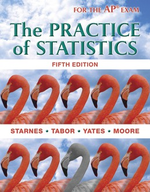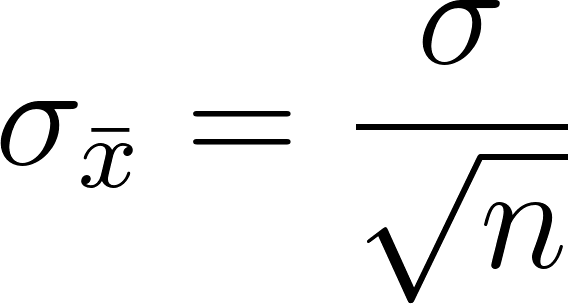×
Get Full Access to The Practice Of Statistics - 5 Edition - Chapter 7.3 - Problem 51
Get Full Access to The Practice Of Statistics - 5 Edition - Chapter 7.3 - Problem 51

×

# Songs on an iPod Refer to Exercise 49. How many songs would you need to sample if youISBN: 9781464108730 488

## Solution for problem 51 Chapter 7.3

The Practice of Statistics | 5th Edition

• Textbook Solutions
• 2901 Step-by-step solutions solved by professors and subject experts
• Get 24/7 help from StudySoup virtual teaching assistantsThe Practice of Statistics | 5th Edition

4 5 1 404 Reviews
25
2
Problem 51

Songs on an iPod Refer to Exercise 49. How many songs would you need to sample if you wanted the standard deviation of the sampling distribution of x to be 30 seconds? Justify your answer.

Step-by-Step Solution:

Step 1 of 2

Given:The standard deviation of the sampling distribution of the sample meanis the population standard deviation divided by the square root of the sample size:Step 2 of 2

##### ISBN: 9781464108730

This full solution covers the following key subjects: . This expansive textbook survival guide covers 44 chapters, and 1409 solutions. The answer to “Songs on an iPod Refer to Exercise 49. How many songs would you need to sample if you wanted the standard deviation of the sampling distribution of x to be 30 seconds? Justify your answer.” is broken down into a number of easy to follow steps, and 35 words. Since the solution to 51 from 7.3 chapter was answered, more than 509 students have viewed the full step-by-step answer. This textbook survival guide was created for the textbook: The Practice of Statistics, edition: 5. The full step-by-step solution to problem: 51 from chapter: 7.3 was answered by , our top Statistics solution expert on 03/19/18, 03:52PM. The Practice of Statistics was written by and is associated to the ISBN: 9781464108730.

## Discover and learn what students are asking

Statistics: Informed Decisions Using Data : Estimating a Population Mean
?The procedure for constructing a t-interval is robust. Explain what this means.

Statistics: Informed Decisions Using Data : Inference about Two Population Standard Deviations
?In Problems 9–14, assume that the populations are normally distributed. Test the given hypothesis. 14. s1 7 s2 at the a = 0.05 level of signi

Statistics: Informed Decisions Using Data : Tests for Independence and the Homogeneity of Proportions
?Political Affiliation In the Sullivan Statistics Survey, respondents were asked to disclose their political affiliation (Democrat, Independent, Republ

Statistics: Informed Decisions Using Data : Testing the Significance of the Least-Squares Regression Model
?In Problems 5–10, use the results of Problems 7–12, respectively, from Section 4.2 to answer the following questions: (a) What are the estima

Unlock Textbook Solution

Enter your email below to unlock your verified solution to:

Songs on an iPod Refer to Exercise 49. How many songs would you need to sample if you